## Star Delta Control Circuit:

The star delta transformation is another technique useful in solving complex networks. Basically, any three circuit elements, i.e. resistive, inductive or capacitive, may be connected in two different ways. One way of connecting these elements is called the star connection, or the Y connection. The other way of connecting these elements is called the delta (Δ) connection. The circuit is said to be in Star Delta Control Circuit, if three elements are connected as shown in Fig. 3.1(a), when it appears like a star (Y). Similarly, the circuit is said to be in delta connection, if three elements are connected as shown in Fig. 3.1(b), when it appears like a delta (Δ).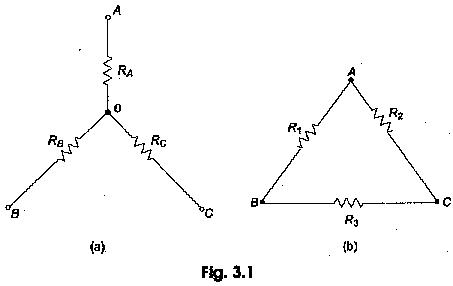The above two circuits are equal if their respective resistances from the terminals AB, BC and CA are equal. Consider the Star Delta Control Circuit in Fig. 3.1(a); the resistance from the terminals AB, BC and CA respectively are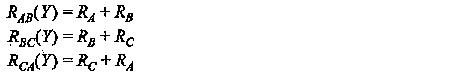Similarly, in the delta connected network in Fig. 3.1(b), the resistances seen from the terminals AB, BC and CA, respectively, are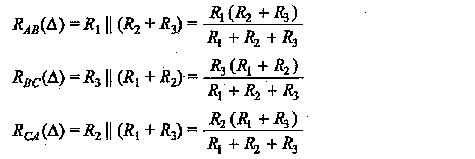Now, if we equate the resistances of Star Delta Control Circuit, we get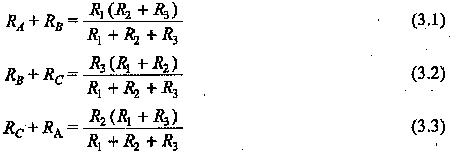Subtracting Eq. 3.2 from Eq. 3.1, and adding Eq. 3.3 to the resultant, we have RI R2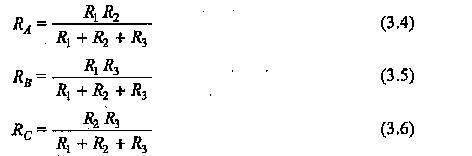Thus, a delta connection of R1, R2 and R3 may be replaced by a star connection of RA, RB and RC as determined from Eqs 3.4, 3.5 and 3.6. Now if we multiply the Eqs 3.4 and 3.5, 3.5 and 3.6, 3.6 and 3.4, and add the three, we get the final equation as under: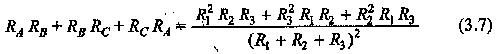In Eq. 3.7 dividing the LHS by RA, gives R3; dividing it by RB gives R2, and doing the same with RC, gives R1.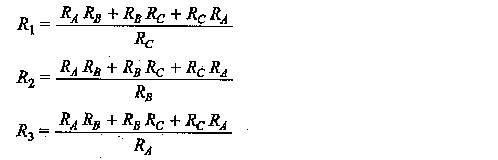From the above results, we can say that a star connected circuit can be transformed into a delta connected circuit and vice-versa.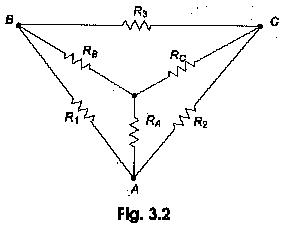From Fig. 3.2 and the above results, we can conclude that any resistance of the delta circuit is equal to the sum of the products of all possible pairs of star resistances divided by the opposite resistance of the star circuit. Similarly, any resistance of the star circuit is equal to the product of two adjacent resistances in the delta connected circuit divided by the sum of all resistances in delta connected circuit.

Scroll to Top